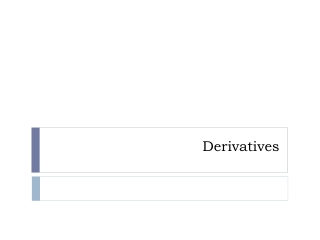DownloadDownload PresentationDerivatives

# Derivatives

Télécharger la présentation## Derivatives

- - - - - - - - - - - - - - - - - - - - - - - - - - - E N D - - - - - - - - - - - - - - - - - - - - - - - - - - -
##### Presentation Transcript

1. Derivatives

2. Constant Rule

3. Power Rule

4. Examples Find the derivatives of each: f(x)=x6 f(x)=x1000

5. Example • Find an equation of a tangent line to the curve at the point (1, 1)

6. Constant Multiple Rule If c is a constant and f is differentiable, then

7. Example: Find derivative of: f(x)=3x4 f(x)= -x

8. Sum Rule If f and g are both differentiable, then

9. Difference Rule If f and g are both differentiable, then

10. Example Find the derivative of